# The identity of: sin^4x+cos^4x= (sin^2x+cos^2x)(sin^2x+cos^2x) sin^2x+cos^2=1 This is the answer I come up with, it is not one of the options available as an answer related homework questions

• #### It’s review question, I need this as soon as possible. Thank you 3) For thè diferential equation: (a) The point zo =-1 is an ordinary point. Compute the recursion formula for the coefficients of...It’s review question, I need this as soon as possible. Thank you 3) For thè diferential equation: (a) The point zo =-1 is an ordinary point. Compute the recursion formula for the coefficients of the power series solution centered at zo- -1 and use it to compute the first three nonzero terms of the power series when -1)-s and v(-1)-0. (25 points) (b) Th...

• #### The identity of: sin^4x+cos^4x= (sin^2x+cos^2x)(sin^2x+cos^2x) sin^2x+cos^2=1 This is the answer I come up with, it is not one of the options available as an answer

The identity of: sin^4x+cos^4x= (sin^2x+cos^2x)(sin^2x+cos^2x) sin^2x+cos^2=1 This is the answer I come up with, it is not one of the options available as an answer. The answers given are 1. -2sin^2xcos^2x 2. 1+2sin^2x-2sin^4x 3. 1+3sin^3x-2sin^2x 4. 1-2sin^2x+2sin^4x 5. 0

• #### Dont copié formé thé book oh ya dont copié formé thé book cause you Oiil inde up being triste soi remembré not toi copié frome thé book oh ya

Dont copié formé thé book oh ya dont copié formé thé book cause you Oiil inde up being triste soi remembré not toi copié frome thé book oh ya!translation in english please!

• #### 8. (a) (5pt) Prove the identity: cscx -cos x=sec x . sin'x 4 and tan θ<0 (b) (5pt) Find cos θ , if sin θ 8. (a) (5pt) Prove the identity: cscx -cos x=sec x . sin'x 4 and tan θ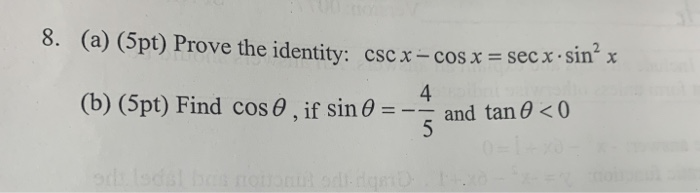8. (a) (5pt) Prove the identity: cscx -cos x=sec x . sin'x 4 and tan θ<0 (b) (5pt) Find cos θ , if sin θ 8. (a) (5pt) Prove the identity: cscx -cos x=sec x . sin'x 4 and tan θ

• #### 2. A dragon is flying around in a pattern given by the parametric curve r(t) (cos(t) cos((sin(t) sin(t) cos(t)j. cos(t) - cos sin(t)-sin(t) cos(t))j (a) Find a formula for the velocity of the dragon...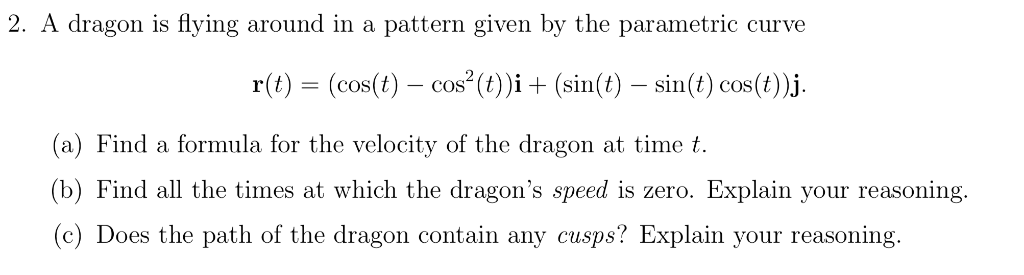2. A dragon is flying around in a pattern given by the parametric curve r(t) (cos(t) cos((sin(t) sin(t) cos(t)j. cos(t) - cos sin(t)-sin(t) cos(t))j (a) Find a formula for the velocity of the dragon at time t (b) Find all the times at which the dragon's speed is zero. Explain your reasoning. c) Does the path of the dragon contain any cusps? Explain your...

• #### The only ideas that can be used include: area ABCI-RA2(A+B+C-lpi), the Pythagorean theorem: Cos c cos a cos b. Vectors-dot product cross product, sin A-sin a/sin c; cos A-cos a sin b/sin c; spher...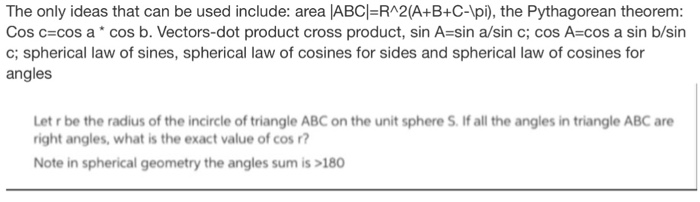The only ideas that can be used include: area ABCI-RA2(A+B+C-lpi), the Pythagorean theorem: Cos c cos a cos b. Vectors-dot product cross product, sin A-sin a/sin c; cos A-cos a sin b/sin c; spherical law of sines, spherical law of cosines for sides and spherical law of cosines for angles Let r be the radius of the incircle of triangle ABC on the unit sp...

• #### sin^2 theta + 2 cos theta -1/ (sin^2 theta +3 cos theta-3= cos^2 theta+cos theta/ (-sin^2 theta) Prove the identity

sin^2 theta + 2 cos theta -1/ (sin^2 theta +3 cos theta-3= cos^2 theta+cos theta/ (-sin^2 theta)Prove the identity.

• #### Solve the following equation for 0 less than and/or equal to "x" less than and/or equal to 360 -- cos^2x - 1 = sin^2x -- Attempt: cos^2x - 1 - sin^2x = 0 cos^2x - 1 - (1 - cos^2x) = 0 cos^2x - 1 - 1 + cos^2x = 0 2cos^2x - 2 = 0 (2cos^2x/2)= (-2/2) cos^

Solve the following equation for 0 less than and/or equal to "x" less than and/or equal to 360--cos^2x - 1 = sin^2x--Attempt:cos^2x - 1 - sin^2x = 0cos^2x - 1 - (1 - cos^2x) = 0cos^2x - 1 - 1 + cos^2x = 02cos^2x - 2 = 0(2cos^2x/2)= (-2/2)cos^2x = -1cosx = square root of -1And I can't do anything with this now...what am I doing wrong?--Textbook answers:0, 180...

• #### convert to radians 5) γ 4cos (2θ-5) r 3 (sin(e - 2))2 + 4 cos(20-5) r-6(sin(9-2))2 + 4 cos(2e-5) 9(sin(9-2))2 + 4 cos(2e-5) r 12(sin(e - 2))2 +4 cos(20 5) 5) γ 4cos (2θ-5) r 3 (sin(e - 2))2 +...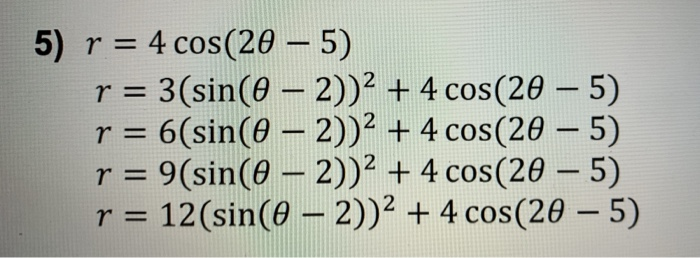convert to radians 5) γ 4cos (2θ-5) r 3 (sin(e - 2))2 + 4 cos(20-5) r-6(sin(9-2))2 + 4 cos(2e-5) 9(sin(9-2))2 + 4 cos(2e-5) r 12(sin(e - 2))2 +4 cos(20 5) 5) γ 4cos (2θ-5) r 3 (sin(e - 2))2 + 4 cos(20-5) r-6(sin(9-2))2 + 4 cos(2e-5) 9(sin(9-2))2 + 4 cos(2e-5) r 12(sin(e - 2))2 +4 cos(20 5)

• #### find all solutions in the interval [0,2 pi) sin(x+(3.14/3) + sin(x- 3.14/3) =1 sin^4 x cos^2 x Since sin (a+b) = sina cosb + cosb sina and sin (a-b) = sina cosb - cosb sina, the first problem can be written 2 sin x cos (pi/3)= sin x The soluti

find all solutions in the interval [0,2 pi) sin(x+(3.14/3) + sin(x- 3.14/3) =1 sin^4 x cos^2 xSince sin (a+b) = sina cosb + cosb sina and sin (a-b) = sina cosb - cosb sina, the first problem can be written 2 sin x cos (pi/3)= sin x The solution to sin x = 1 is x = pi/2 For your other problem sin^4 cos^2 x = sin^4 x(1 - sin^2x)=0 The solutions are sin x = 0 a...

• #### Options for question 1) Work Hard/Shirk Options for question 2) Are/Are Not Options for question ...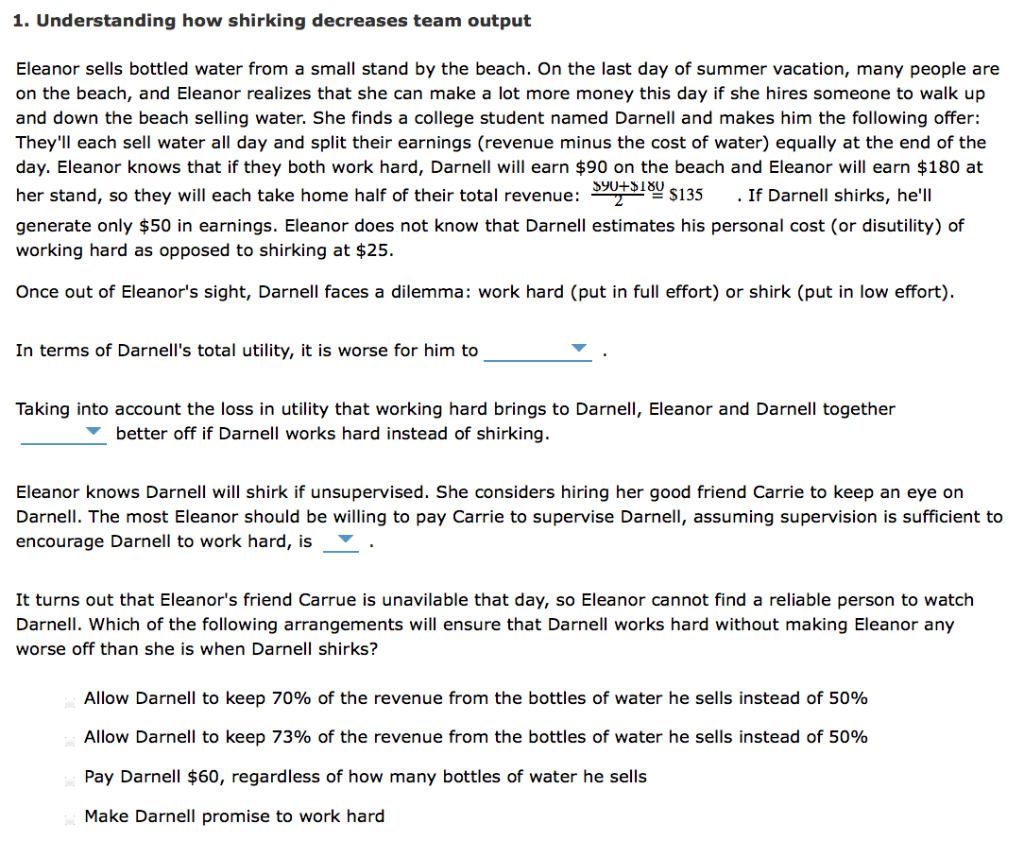Options for question 1) Work Hard/Shirk Options for question 2) Are/Are Not Options for question 3) \$45, \$25,\$15,\$20 1. Understanding how shirking decreases team output Eleanor sells bottled water from a small stand by the beach. On the last day of summer vacation, many people are on the beach, and Eleanor realizes that she can make a lot more money this da...

• #### How would you establish this identity: (1+sec(beta))/(sec(beta))=(sin^2(beta))/(1-cos(beta)) on the right, sin^2 = 1-cos^2, that factor to 1-cos * `1+cos, then the denominator makes the entire right side 1+cosB which is 1+1/sec which is 1/sec (sec+1)

How would you establish this identity: (1+sec(beta))/(sec(beta))=(sin^2(beta))/(1-cos(beta))on the right, sin^2 = 1-cos^2, that factor to 1-cos * `1+cos, then the denominator makes the entire right side 1+cosB which is 1+1/sec which is 1/sec (sec+1) qedusing sec(beta) = 1/cos(beta): 1+sec(beta))/(sec(beta))= 1 + cos(beta) sin^2(beta)/(1-cos(beta)) = (1-cos^2...

• #### verify the following identity used in calculus: cos(x+h)-cos(x)/h=cos(x)[cos(h)-1/h]-sin(x)[sin(h)/h]

verify the following identity used in calculus: cos(x+h)-cos(x)/h=cos(x)[cos(h)-1/h]-sin(x)[sin(h)/h]

• #### Help With VBA Coding User options OK Select one of the following options: Options Cancel Find an optimal production plan C View demand and cost data Enter new demand and cost data C Change smoothing...Help With VBA Coding User options OK Select one of the following options: Options Cancel Find an optimal production plan C View demand and cost data Enter new demand and cost data C Change smoothing constants New Data Enter the newly observed demand and Add Data unit cost for Feb-2006 Demand Unit cost: Screenshot of "New Data" dialog box New Data En...

• #### verify that each of the following is an identity: 1) cos x/ 1-sin^2x= sec x 2) sec x/sin x - sin x/cos x = cot x 3) 1+tan^2ø/ cos ^2ø = sec^4 ø I really need ur help :) thank u so much

verify that each of the following is an identity: 1) cos x/ 1-sin^2x= sec x 2) sec x/sin x - sin x/cos x = cot x 3) 1+tan^2ø/ cos ^2ø = sec^4 ø I really need ur help :) thank u so much

• #### the original problem was: (sin x + cos x)^2 + (sin x - cos x)^2 = 2 steps too please I got 1 for (sin x + cos x)^2 but then what does (sin x - cos x)^2 become since it's minus

the original problem was: (sin x + cos x)^2 + (sin x - cos x)^2 = 2steps too pleaseI got 1 for (sin x + cos x)^2 but then what does (sin x - cos x)^2 become since it's minus?

• #### 2) Use the sum and difference identites sin[x + pi/4] + sin[x-pi/4] = -1 sinx cospi/4 + cosxsin pi/4 + sinx cos pi/4 - cosx sin pi/4 = -1 2 sin x cos pi/4 =-1 cos pi/4 = sqr2/2 2sin^x(sqrt2/2) = -1 sin x = -sqrt2 x = 7pi/4 and 5pi/4 Am I co

2) Use the sum and difference identites sin[x + pi/4] + sin[x-pi/4] = -1 sinx cospi/4 + cosxsin pi/4 + sinx cos pi/4 - cosx sin pi/4 = -1 2 sin x cos pi/4 =-1 cos pi/4 = sqr2/2 2sin^x(sqrt2/2) = -1 sin x = -sqrt2 x = 7pi/4 and 5pi/4 Am I correct?

• #### cos(tan + cot) = csc only simplify one side to equal csc so far I got this far: [((cos)(sin))/(cos)] + [((cos)(cos))/(sin)] = csc I don't know what to do next

cos(tan + cot) = csconly simplify one side to equal cscso far I got this far: [((cos)(sin))/(cos)] + [((cos)(cos))/(sin)] = cscI don't know what to do next

• #### ANSWER 3: Deduction:-14.285714285714390 -300e 800t cos (600t) 384e-800f sin(600t) mA cos (600t) 192e-800f sin(600t) mA -300e 800t cos (600t) - 192e-800f sin(600t) mA -600e 800t cos (600t) 192e-800f s...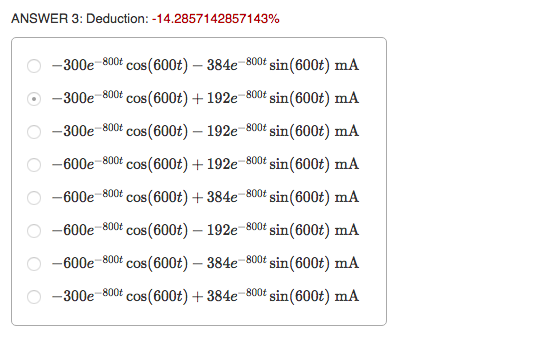ANSWER 3: Deduction:-14.285714285714390 -300e 800t cos (600t) 384e-800f sin(600t) mA cos (600t) 192e-800f sin(600t) mA -300e 800t cos (600t) - 192e-800f sin(600t) mA -600e 800t cos (600t) 192e-800f sin(600t) mA -600e 800t cos(600t) 384e-800f sin(600t) mA -600e 800t cos (600t) - 192e-800f sin(600t) mA -600e 800t cos (600t) 384e-800f sin(600t) mA -300e 800t c...

• #### #40 a-f B-A. (B+A ". Beats slation Recall the identity cos A-cos Be2-2A)sin(-2A) a. Show that 0-10,a, . 9 and (ii)o_10,us2toverify the identity. In which case do you see Gaph the functions on...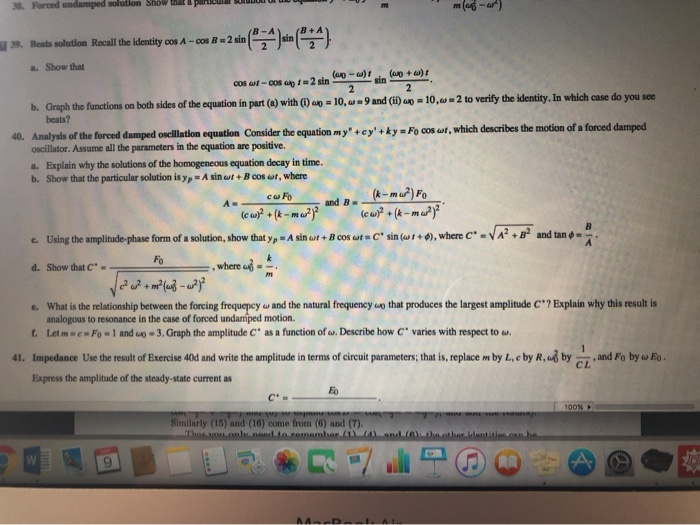#40 a-f B-A. (B+A ". Beats slation Recall the identity cos A-cos Be2-2A)sin(-2A) a. Show that 0-10,a, . 9 and (ii)o_10,us2toverify the identity. In which case do you see Gaph the functions on both sides of the equation in part (a) with (i) beats? b. 40 Analysis of the forced damped oscillation equation Consider the equation my"+ey'+ky Fo cos...